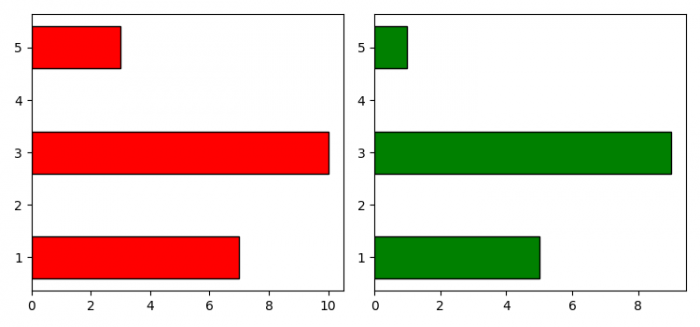# Plot two horizontal bar charts sharing the same Y-axis in Python Matplotlib

To plot two horizontal bar charts sharing the same Y-axis, we can use sharey=ax1 in subplot() method and for horizontal bar, we can use barh() method.

## Steps

• Create lists for data points.
• Create a new figure or activate an existing figure using figure() method
• Add a subplot to the current figure using subplot() method, at index=1.
• Plot horizontal bar on axis 1 using barh() method.
• Add a subplot to the current figure using subplot() method, at index=2. Share the Yaxis of axis 1.
• Plot the horizontal bar on axis 2.
• To display the figure, use show() method.

## Example

import matplotlib.pyplot as plt
import numpy as np

plt.rcParams["figure.figsize"] = [7.50, 3.50]
plt.rcParams["figure.autolayout"] = True

y = [3, 1, 5]
x1 = [10, 7, 3]
x2 = [9, 5, 1]

fig = plt.figure()

axe1 = plt.subplot(121)
axe1.barh(y, x1, align='center', color='red', edgecolor='black')

axe2 = plt.subplot(122, sharey=axe1)
axe2.barh(y, x2, align='center', color='green', edgecolor='black')

plt.show()

## Output